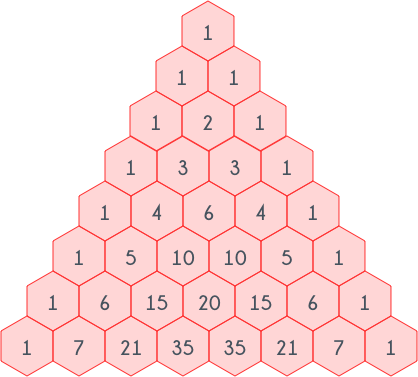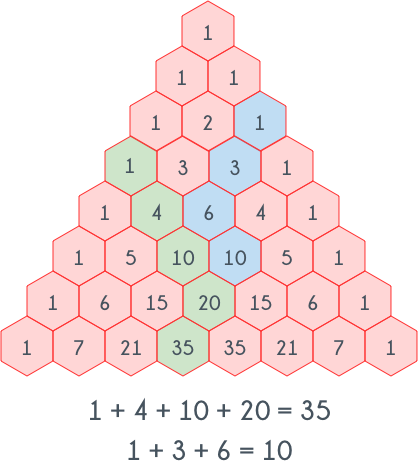Math
Topics:
Basics of Combinatorics

# Basics of Combinatorics

Combinatorics is all about number of ways of choosing some objects out of a collection and/or number of ways of their arrangement. For example suppose there are five members in a club, let's say there names are A, B, C, D, and E, and one of them is to be chosen as the coordinator. Clearly any one out of them can be chosen so there are 5 ways. Now suppose two members are to be chosen for the position of coordinator and co-coordinator. Now, we can choose A as coordinator and one out of the rest 4 as co-coordinator. Similarly we can choose B as coordinator and one of out the remaining 4 as co-coordinator, and similarly with C, D and E. So there will be total 20 possible ways.

Note that in the previous example choosing A then B and choosing B then A, are considered different, i.e. the way of arrangement matter. Now suppose two coordinators are to be chosen, so here choosing A, then B and choosing B then A will be same. Number of different ways here will be 10.

In the first example we have to find permutation of choosing 2 members out of 5 and in the second one we have to find out combination of choosing 2 members out of 5.

Let's generalize it. Permutations of choosing $R$ disticnt objects out of a collection of $N$ objects can be calculated using the following formula: $$^NP_R = \frac{N!}{(N-R)!}$$ Combinations of choosing $R$ distinct objects out of a collection of $N$ objects can be calculated using the following formula: $$^NC_R = \frac{N!}{(N-R)! \times R!}$$

## Basic Combinatorics Rules:

Suppose there are two sets $A$ and $B$.

The basic rules of combinatorics one must remember are:

1. The Rule of Product:
The product rule states that if there are $X$ number of ways to choose one element from $A$ and $Y$ number of ways to choose one element from $B$, then there will be $X \times Y$ number of ways to choose two elements, one from $A$ and one from $B$.

2. The Rule of Sum:
The sum rule states that if there are $X$ number of ways to choose one element from $A$ and $Y$ number of ways to choose one element from $B$, then there will be $X+Y$ number of ways to choose one element that can belong to either $A$ or to $B$.

These rules can be used for a finite collections of sets.

Permutations with repetition:

If we have $N$ objects out of which $N_1$ objects are of type $1$, $N_2$ objects are of type $2$, ... $N_k$ objects are of type $k$, then number of ways of arrangement of these $N$ objects are given by:

$$\frac{N!}{N_1!N_2!...N_k!}$$

Combinations with repetition:

If we have $N$ elements out of which we want to choose $K$ elements and it is allowed to choose one element more than once, then number of ways are given by: $$^{N+K-1}C_K = \frac{(N+K-1)!}{(K)!(N-1)!}$$

## Pascal Triangle:

The image given below shows a pascal triangle.As can be seen in the $i^{th}$ row there are $i$ elements, where $i \ge 1$. $j^{th}$ element of $i^{th}$ row is equal to $^{i-1}C_{j-1}$ where $1 \le j \le i$.

There are several interesting properties in Pascal triangle.
The corner elements of each row are always equal to 1($^{i-1}C_0$ and $^{i-1}C_{i-1}$, $i \ge 1$). All the other $(i, j)^{th}$ elements of the triangle, (where $i \ge 3$ and $2 \le j \le i-1$) , are equal to the sum of $(i-1,j-1)^{th}$ and $(i-1,j)^{th}$ element. So, because of this property, a dynamic programming approach can be used for computing pascal triangle. Following is the pseudo code for that.


function pascal_triangle(MAXN)
intialize a matrix dp[MAXN][MAXN] with 0
for i = 0 to MAXN
dp[i]=dp[i]=1
endfor
for i = 1 to MAXN
for j = 1 to MAXN
dp[i][j] = dp[i-1][j]+dp[i][j-1]
endfor
endfor



In the code given above $dp[i][j]$ denotes $^{i+j}C_{i}$
Another interesting property of pascal triangle is, the sum of all the elements in $i^{th}$ row is equal to $2^{i-1}$, where $i \ge 1$.

Hockey Stick Rule:
Hockey sticky rule is simply the equality given below:
$$\sum_{i=0}^{r} {^{n+i}C_i} = \sum_{i=0}^{r} {^{n+i}C_n} = ^{n+r+1}C_{r} = ^{n+r+1}C_{n+1}$$ The following image will make it more clear.Now let's try to solve the problem given below.
Given $N$ and $K$ find out how many different ways are there to represent $N$ as sum of $K$ non-zero integers.
Let's take an example. Sum of elements of following sets, which are of size 3, is equal to 5:
$\{1, 1, 3 \}$
$\{1, 3, 1\}$
$\{3, 1, 1\}$
$\{2, 2, 1\}$
$\{2, 1, 2\}$
$\{1, 2, 2\}$

We can rewrite the above sets as follows:
$\{1, 1, 1+1+1 \}$
$\{1, 1+1+1, 1\}$
$\{1+1+1, 1, 1\}$
$\{1+1, 1+1, 1\}$
$\{1+1, 1, 1+1\}$
$\{1, 1+1, 1+1\}$

So, clearly there are exactly five $1's$, and between those there is either a comma or a plus sign, and also comma appears exactly 2 times.
$\{1 - 1 - 1 - 1 - 1\}$
Clearly there are 4 dashes and we have to choose 2 out of those and place a comma there, and at the rest place plus sign. So, number of way of choosing 2 objects out of 4 is $^4C_2 = 6$.
In general, for $N$ there will be $N-1$ dashes, and out of those we want to choose $K-1$ and place comma in place of those and in place of rest of the dashes place plus sign. So ways of choosing $K-1$ objects out of $N-1$ is $^{N-1}C_{K-1}$

Contributed by: Vaibhav Jaimini